Published: 30 September 2021

# Mathematical simulation of adaptive vector finite element method for the analysis of electromagnetic vibration spectrum field response

Yiyuan Cheng1
Mingyang Su2
Ming Hui3
Wei Liu4
Yangbing Zheng5
1, 4, 5Department of Mechanical and Electrical Engineering, Nanyang Normal University, Nanyang, Henan, 473061, China
2Department of Environmental Engineering, Henan Polytechnic Institute, Nanyang, Henan, 473000, China
3Department of physics and Electronic Engineering, Nanyang Normal University, Nanyang, Henan, 473061, China
Corresponding Author:
Yiyuan Cheng
Views 67

#### Abstract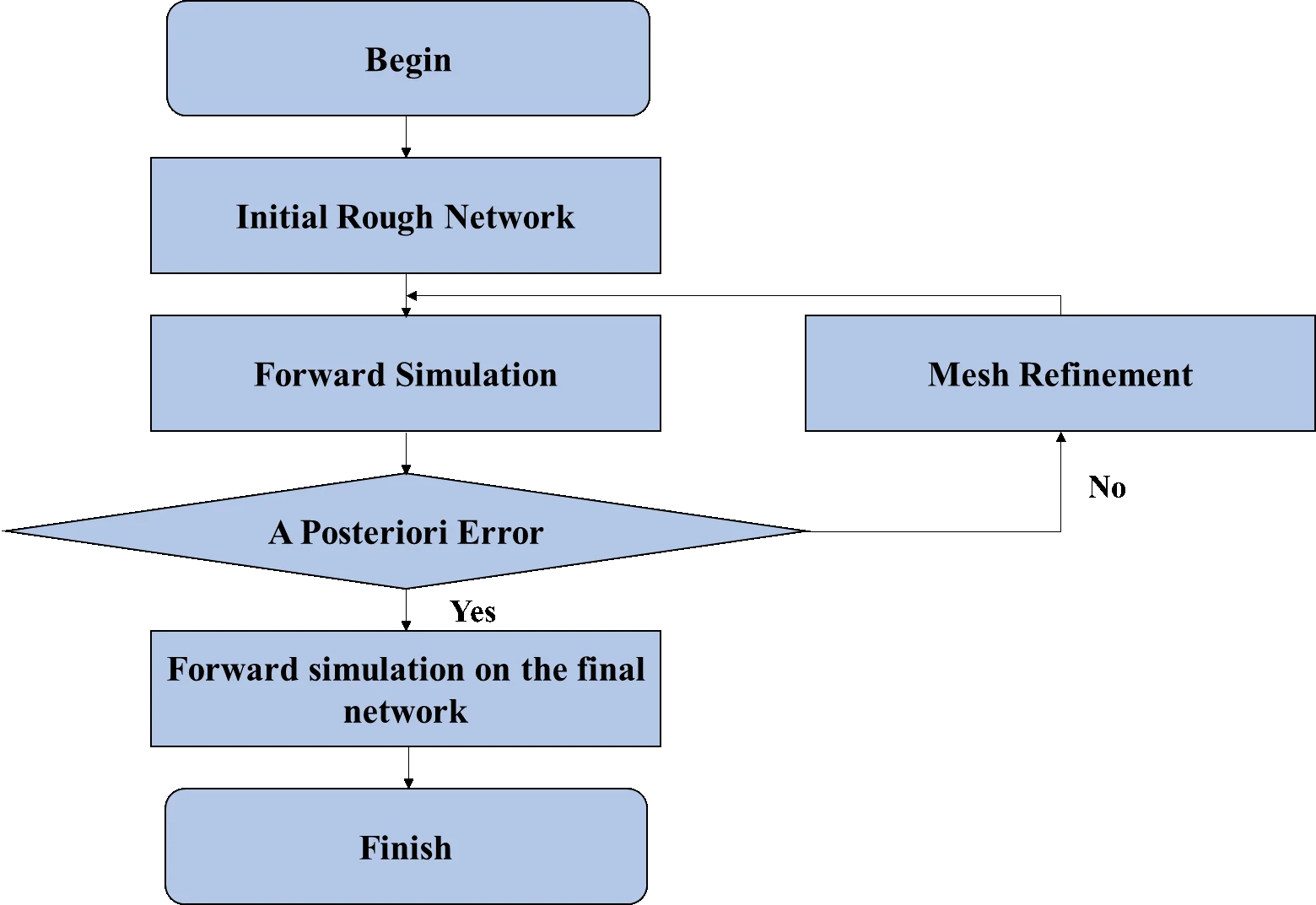#### Highlights

• The three-dimensional positive calculation of controlled source electromagnetic vibration spectrum field is realised based on the finite meta-method utilizing the non-structural mesh vector and adaptive mesh refinement technology.
• The 3D meshing for the simulation of terrain fluctuations and complex electrical anomalies is addressed in this article by proposing a three-dimensional adaptive vector finite meta-acting algorithm of controlled source electromagnetism (CSEM).
• An adaptive vector finite element algorithm is used to realize the CSEM3D forward simulation and the primary/secondary field separation algorithms are utilized for the calculation of the electromagnetic vibration spectrum field response.
• The controlled source electromagnetic response with three-dimensional tilt plate abnormality is simulated by using this algorithm.

## 1. Introduction

In the exploration of ground electromagnetic vibration spectrum method, controllable source audio geomagneance method (CSAMT) plays an important role in the survey and exploration of petroleum, geothermal, metal mineral, hydration, environment and so on. Ocean controlled source electromagnetic vibration spectrum method (CSEM) is a new method of marine geophysical exploration, which can help seismic methods to identify effective reservoirs and improve drilling success rate. Marine CSEM technology has been widely used in the exploration of marine oil and gas resources, and has achieved remarkable results. In controllable source electromagnetic vibration spectrum method, the source, field and observation system are all three-dimensional in essence, and solving the three-dimensional problem is the most important way to improve the reliability and resolution of data [1-3].

This paper realizes the three-dimensional positive calculation of controlled source electromagnetic vibration spectrum field based on the finite meta-method utilizing the non-structural mesh vector and adaptive mesh refinement technology [18-20]. The 3D meshing for the simulation of terrain fluctuations and complex electrical anomalies is addressed in this article by proposing a three-dimensional adaptive vector finite meta-acting algorithm of controlled source electromagnetic vibration spectrum (CSEM) field. An adaptive vector finite element algorithm is used to realize the CSEM3D forward simulation and the primary/secondary field separation algorithms are utilized for the calculation of the electromagnetic vibration spectrum field response. The controlled source electromagnetic response with three-dimensional tilt plate abnormality is simulated by using this algorithm and the numerical solutions of analytical solution and three-dimensional finite volume method are compared and analysed. The outcomes obtained reveals the ability and effectiveness of the algorithm to simulate electromagnetic vibration spectrum field of complex geoelectrical structure [21-23]. The algorithms utilized in this article can calculate the electromagnetic field of a galvanic source with a finite length in any posture, and the source and receiving points can be placed in any position, providing good versatility.

The rest of this article is organised as: The description of unstructured grid vector finite element method is given in Section 2 followed by the explanation of adaptive mesh refinement algorithm based on posterior error estimation in Section 3. Results and analysis of the numerical simulation are presented in Section 4 which is trailed by the concluding remarks in Section 5.

## 2. Unstructured grid vector finite element method

### 2.1. Governing equation

Assuming the time factor is ${e}^{-i\omega t}$, in the quasi-steady state, the governing equations satisfied by the electric field ($E$) and the magnetic field ($H$) are:

1
$\begin{array}{l}\nabla ×E-i\omega {\mu }_{0}H=0,\\ \nabla ×H-\sigma E=J,\end{array}$

where ${\mu }_{0}$ is the magnetic permeability in vacuum, $\omega$ is the angular frequency, $J$ is a current source, $\sigma$ is the conductivity. In order to eliminate the singularity of the electromagnetic vibration spectrum field near the source point, the electromagnetic vibration spectrum field is decomposed into ${\sigma }^{p}$ the primary field (${E}^{p}$ with ${H}^{p}$) and conductivity ${\sigma }^{s}=\sigma -{\sigma }^{p}$ the secondary field produced by the anomalous body (${E}^{s}$ with ${H}^{s}$). The primary field can be obtained by the pseudo-analytical method [24-26].

The secondary electromagnetic vibration spectrum field satisfies the following equation:

2
$\begin{array}{l}\nabla ×{E}^{s}-i\omega {\mu }_{0}{H}^{s}=0,\\ \nabla ×{H}^{s}-\sigma {E}^{s}={\sigma }^{s}{E}^{p}.\end{array}$

From Eq. (2), it can be deduced that the secondary electric field ${E}^{s}$ partial differential equation:

3
$\nabla ×\nabla ×{E}^{s}-i\omega {\mu }_{0}{E}^{s}=i\omega {\mu }_{0}{\sigma }^{s}{E}^{p}.$

We use the finite element method to solve Eq. (3). When the secondary electric field is obtained ${E}^{s}$ after that, the secondary magnetic field can be calculated using Eq. (4):

4
${H}^{s}=\frac{\nabla ×{E}^{s}}{i\omega {\mu }_{0}}.$

### 2.2. Finite element analysis

Using the weighted residual method, the integral equation corresponding to the partial differential Eq. (3) can be derived. Multiply the left and right sides of Eq. (3) by any variation of the electric field $\delta {E}^{s}$, and integrate the simulation area $\mathrm{\Omega }$, combined with the divergence formula and green's theorem, we can get:

5
$\begin{array}{c}{\int }_{\mathrm{\Omega }}\left[\left(\nabla ×\delta {E}^{s}\right)\cdot \left(\nabla ×{E}^{s}\right)-\delta {E}^{s}\cdot i\omega {\mu }_{0}\sigma {E}^{s}\right]d\mathrm{\Omega }={\int }_{\mathrm{\Omega }}i\omega {\mu }_{0}\sigma {E}^{s}{\sigma }^{s}{E}^{p}d\mathrm{\Omega }.\end{array}$

Assume that the secondary electric field satisfies the homogeneous boundary condition on the outer boundary of the simulation area:

6
$n×{E}^{s}=0.$

The finite element Eq. (5) can be solved by the conventional nodal finite element method and the vector finite element method, but when the nodal finite element method is used, the continuous boundary condition of the normal component of the electric field and the condition that the electromagnetic vibration spectrum field divergence is zero cannot be guaranteed. As a result, false solutions may appear, and the processing of curl operators and the application of boundary conditions are more troublesome. Eq. (5) can be obtained through a certain transformation to obtain the electromagnetic vibration spectrum potential equation under the coulomb gauge or the Lorentz gauge. Solving the electromagnetic vibration spectrum potential avoids the normal discontinuity of the electric field in the earth's medium. It can be solved directly by the nodal finite element method. However, because the interpolation function of the nodal finite element does not satisfy the condition of zero divergence, a false solution will be generated, and the accuracy of the numerical simulation will obviously be reduced when the electromagnetic vibration spectrum potential is solved first and then the electromagnetic vibration spectrum field is solved by the difference scheme. The vector finite element method can overcome the above problems [27, 28].

Fig. 1Schematic diagram of tetrahedral element nodes and vector edges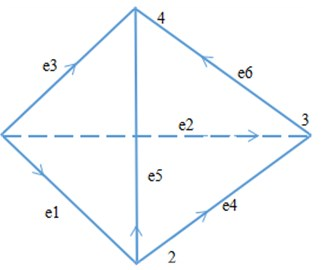We use unstructured tetrahedral elements to discretize the simulation area. Fig. 1 shows a tetrahedral element with 4 nodes and 6 edges. Assume that the tangential component of the electric field on the edge is ${E}_{i}\left(i=1,...,6\right)$, and the direction of the tangential component of the electric field at the midpoint of the edge is consistent with the direction of the edge. The electric field at any point in the unit can be obtained by linear interpolation of the electric field on the 6 edges in the unit:

7
$E={\sum }_{i=1}^{6}{N}_{i}{E}_{i},$

where ${N}_{i}$ it is a linear interpolation basis function. We use nédélec type vector basis functions:

8
${N}_{i}={l}_{i}\left({L}_{i1}\nabla {L}_{i2}-{L}_{i2}\nabla {L}_{i1}\right),$

where ${l}_{i}$ is the length of the $i$th side, ${L}_{i1}$, ${L}_{i2}$ are the starting points of the $i$th edge ${i}_{1}$ and the end ${i}_{2}$ the node basis function. Correct ${N}_{i}$ take the divergence, get $\nabla \cdot {N}_{i}=0$, that is, the nédélec-type vector basis function satisfies the condition of zero divergence .

Substituting Eq. (7) into integral Eq. (5), and calculating the unit integral, we can get:

9
$\delta {E}^{s}\left({\sum }_{1}^{ne}{K}_{1}^{e}+{K}_{2}^{e}\right){E}^{s}=\delta {E}^{s}{\sum }_{1}^{ne}{p}^{e},$

among them, $ne$ is the number of tetrahedral units:

10
${K}_{1}^{e}={\int }_{{\mathrm{\Omega }}_{e}}\left[{\left(\nabla ×N\right)}^{T}\cdot \left(\nabla ×N\right)\right]d\mathrm{\Omega },$
11
${K}_{2}^{e}=-i\omega \mu \sigma {\int }_{{\mathrm{\Omega }}_{e}}\left[{N}^{T}\cdot N\right]d\mathrm{\Omega },$
12
${P}_{}^{e}=-i\omega {\mu }_{0}{\sigma }^{s}{\int }_{{\mathrm{\Omega }}_{e}}\left[{N}^{T}\cdot {E}^{p}\right]d\mathrm{\Omega }.$

Consider the variation $\delta {E}^{s}$ the arbitrariness of, the finite element linear equations can be obtained from Eq. (9), and the linear equations can be solved by the direct method to obtain the quadratic field, and then add to the primary field vector to obtain the total electromagnetic vibration spectrum field.

## 3. Adaptive mesh refinement algorithm based on posterior error estimation

Eq. (5) can be expressed as:

13
$B\left(u,v\right)=F\left(v\right),$

among them:

14
$B\left(u,v\right)=\int {\mathrm{}}_{\mathrm{\Omega }}\left[\left(\nabla ×u\right)\cdot \left(\nabla ×v\right)-{k}^{2}uv\right]d\mathrm{\Omega },$
$F\left(v\right)=\int {\mathrm{}}_{\mathrm{\Omega }}i\omega \mu {\sigma }^{s}{E}^{p}vd\mathrm{\Omega },$

where ${k}^{2}=i\omega \mu \sigma$.

Assume that the function $G$ is the exact solution u of the electromagnetic vibration spectrum field and the finite element numerical solution ${u}_{h}$ error of $u-{u}_{h}$ the functional of Eq. (13) is:

15
${B}^{*}\left(w,v\right)=G\left(v\right),$

among them, ${B}^{*}$ it is a dual or adjoint operator. Assume ${B}^{*}\left(w,v\right)=B\left(v,w\right)$, then there are:

16
$G\left(u-{u}_{h}\right)={B}^{*}\left(w,u-{u}_{h}\right)=B\left(u-{u}_{h},w\right)=B\left(u-{u}_{h},w-{w}_{h}\right),$

where $w$ and ${w}_{h}$ they are the exact solution of the dual problem and the finite element numerical solution.

According to Eq. (14), the right term of dual Eq. (15) is:

17
$G\left(v\right)=\frac{1}{{‖\nabla ×u‖}_{{L}^{2}\left(\mathrm{\Omega }\right)}^{2}}\left(\int {\mathrm{}}_{\mathrm{\Omega }}\left(f+{k}^{2}u\right)\cdot v-\left(\nabla ×u\right)×\left(\nabla ×v\right)d\mathrm{\Omega }$
$-\int {\mathrm{}}_{\partial \mathrm{\Omega }}\left(\left(\nabla ×u\right)×n\right)\cdot vdS.$

Among them, $\mathrm{\Omega }$ is the continuous area containing the receiving point, $\partial \mathrm{\Omega }$ is the boundary of the continuous region, and $v$ is an arbitrary vector. Solving Eq. (15) can obtain the finite element solution of the dual problem ${\omega }_{h}$.

Define a posterior error estimate based on residuals and dual weighting:

18
$\eta ={\eta }_{\tau }\left(u\right){\eta }_{\tau }\left(w\right),$

among them:

19
${\eta }_{\tau }^{2}\left(u\right)={h}^{2}\left({‖{R}_{1}\left(u\right)‖}^{2}+{‖{R}_{2}\left(u\right)‖}^{2}\right)+h{\sum }_{f\in \mathrm{\Omega }}\left({‖{J}_{1}\left(u\right)‖}^{2}+{‖{J}_{2}\left(u\right)‖}^{2}\right),$
20
$\begin{array}{l}{R}_{1}\left(u\right)=\left(f+{k}^{2}u-\left(\nabla ×\nabla ×u\right)\right),\\ {R}_{2}\left(u\right)=\nabla \cdot \left(f+{k}^{2}u\right),\\ {J}_{1}\left(u\right)=\left(\nabla ×u\right)×n,\\ {J}_{2}\left(u\right)=\left(f+{k}^{2}u\right)\cdot n.\end{array}$

In the formula, $h$ is the diameter of the circumcircle of the tetrahedron. ${\eta }_{\tau }\left(w\right)$ calculation formula and ${\eta }_{\tau }\left(u\right)$ similar.

Fig. 2The calculation process of the adaptive finite element algorithm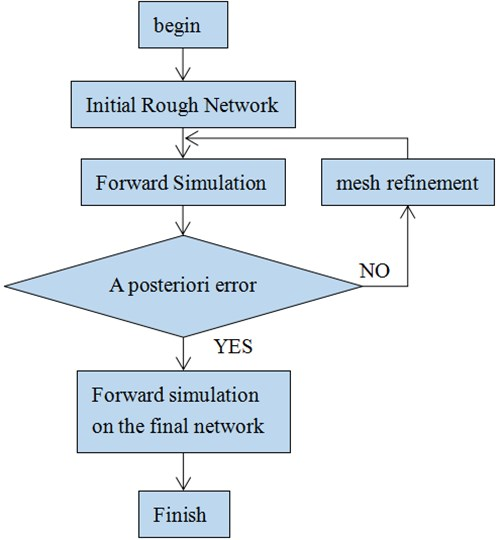The steps of the adaptive finite element algorithm are as follows:

1. Given an initial coarse mesh, use the finite element method to solve Eq. (13) to obtain its finite element solution.

2. Solve the dual problem finite element Eq. (15), obtain the finite element solution to the dual problem.

3. Calculate the posterior error of each element according to Eq. (18), and refine the elements with a large error in a certain proportion (the calculation examples in this paper are set to 5 % of the total number of elements). Get a new mesh.

4. Repeat steps (1)-(3) until the root mean square error of the finite element solution before and after mesh refinement meets the accuracy requirements or reaches the set maximum mesh refinement times. The specific process is shown in Fig. 2 .

## 4. Results and analysis of the numerical calculation

### 4.1. One-dimensional oil and gas model

In order to verify the correctness of the algorithm and program, the 1D model shown in Fig. 3 is designed. Assume that the seawater resistivity is 0.3 Ωm and the seawater depth is 1000 m; the bottom of the seabed is a 1000 m thick overburden with a resistivity of 1 Ωm; the bottom of the overburden is A 500 m thick high-resistance oil and gas layer with a resistivity of 100 Ωm; below the oil and gas layer is a formation with a resistivity of 1 Ωm; the galvanic source is arranged 50 m above the seabed, and its center point is (0 m, 0 m, 950 m), and the length it is 1m, the emission current is 1 A, and the emission frequency is 0.25 Hz. Assume that a total of 20 receiving points are along the survey line direction ($X=$0), and are evenly arranged on the seabed at equal intervals within the range of $Y=$500-10000 m .

Fig. 31D oil and gas model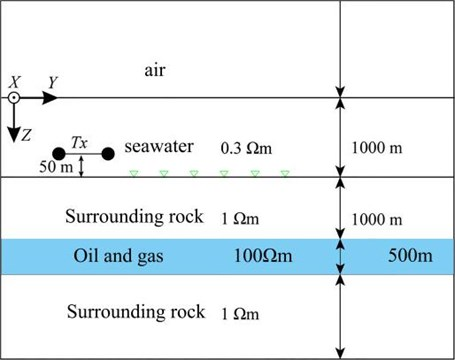Fig. 4 shows the comparison between the adaptive finite element solution and the 1D analytical solution.

The corresponding curve in the figure is the result of the source being arranged in the $X$ direction, and the corresponding curve is the result of the source being arranged in the $Y$ direction. It can be seen that the relative amplitude error of the horizontal electromagnetic vibration spectrum field component is less than 1 %, and the phase difference is within 1°. For the vertical electromagnetic vibration spectrum field component, when the transmission distance is small (less than 5 km), the relative amplitude error is less than 1 %, and the phase difference is 1° when the transmission distance is greater than 5 km, the relative error of the amplitude is relatively large, and the maximum phase difference of the components is 1.4°.

The finite element simulation area is 60 km×60 km×60 km, the initial grid is shown in Fig. 5(a), and the grid of the part of interest in the grid is extracted (Fig. 5(b)) (0 km $1 km, –0.5 km $ 10.5 km, –0.5 km $ 3 km), it can be seen that the initial grid is very rough, and the grids of the transmitting source and receiving points are not refined.

Fig. 4Comparison of adaptive finite element solution and analytical solution (1D Oil and Gas Model)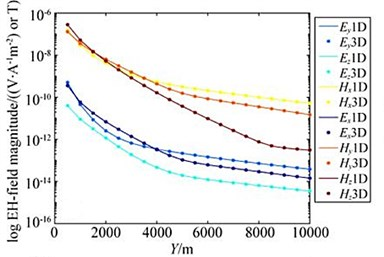(a)(b)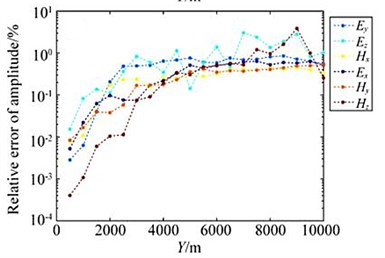(c)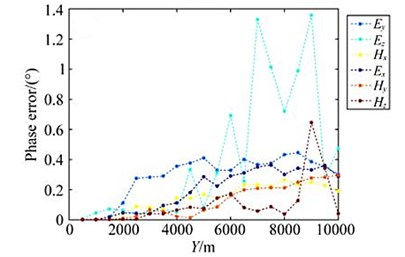(d)

Fig. 5Grid schematic (1D oil and gas model)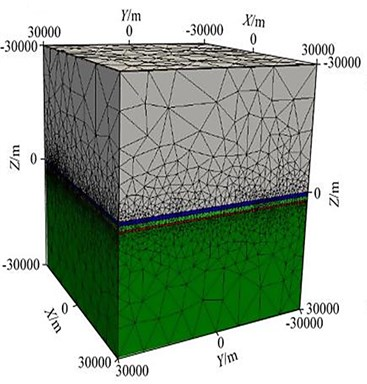a)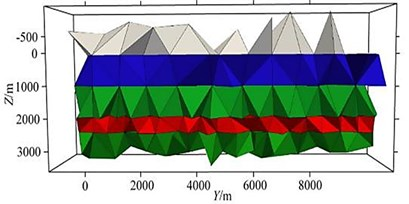b)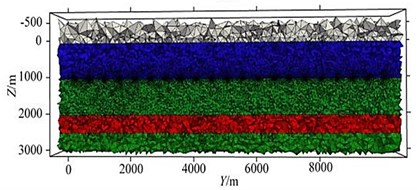c)

After 10 times of mesh refinement, the final mesh obtained is shown in Fig. 5(c). It can be seen from the figure that in the area near the receiving point, the grid is well encrypted, and the grid below the receiving point is also refined to a certain extent, thus ensuring the accuracy of the numerical solution. In the finite element numerical simulation, if you want to obtain a high-precision numerical solution, the finite element mesh needs to be discretized sufficiently fine. The adaptive mesh refinement algorithm uses the posterior error estimation method to estimate the accuracy of the finite element numerical solution, and gradually refines the mesh with larger errors, thereby ensuring the rationality of the mesh refinement. Each time the number of meshes involved in the refinement cannot be too small, too small will require more refinement times; but not too much, too much will also refine the meshes with small errors, thereby increasing calculation amount. After many simulation tests, some units with larger errors (5 % of the total number of units) are refined, and the effect is the best. Fig. 6 shows the change of the relative error of the electromagnetic vibration spectrum field amplitude during the adaptive mesh refinement process.

Fig. 6Changes of relative error of electromagnetic vibration spectrum field amplitude during adaptive mesh refinement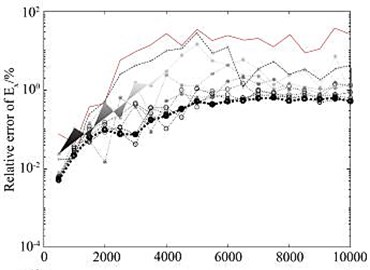a)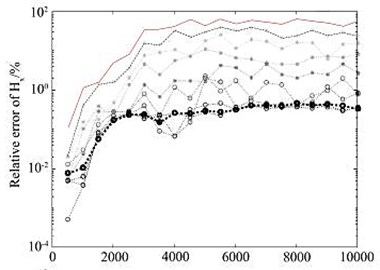b)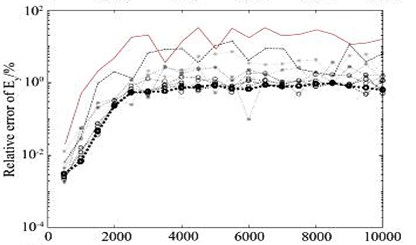c)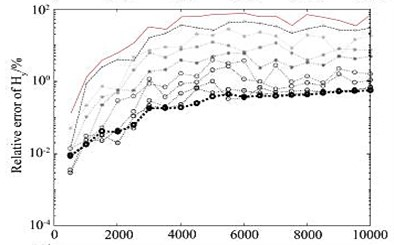d)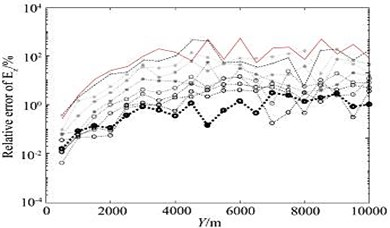e)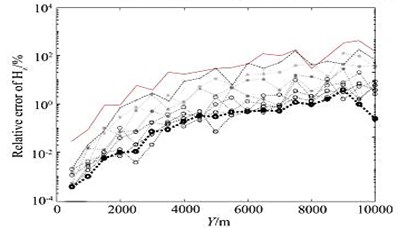f)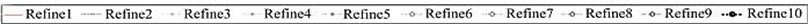It can be seen from the figure that the relative error of the numerical solution amplitude obtained on the initial grid (part of the area shown in Fig. 5(b)) is basically more than 10 %, but as the number of refinements increases, the relative error of the electromagnetic vibration spectrum field amplitude gradually decreases, after 10 times after the mesh is refined (part of the area is shown in Fig. 5(c)), the relative error of the amplitude basically converges to about 1 %.

In the mesh refinement process of the above calculation example, it is theoretically required to refine only 5 % of the total number of cells each time. However, because the meshing software is based on the delaunay meshing algorithm, it may be refined each time the number of grid cells is greater than 5 %. Table 1 details the number of grid cells, the number of edges, CPU time, solver time, memory consumption, primary field time and RmS during the mesh refinement process. Among them, RmS is the root mean square error between the calculation result of each iteration and the calculation result of the previous iteration. It can be seen from the table that when the number of grids is small, the calculation time of one field accounts for the main part, but when the number of grids becomes more and more when it is large, the calculation time required to solve the linear equations becomes longer and longer. After 10 iterations, RmS converges to 1.0 .

### 4.2. Three-dimensional oil and gas model

Establish a three-dimensional ocean oil and gas reservoir model as shown in Fig. 7.

Assume that the seawater resistivity is 0.3 Ωm and the seawater depth is 1000 m; there is a 100 m thick high-resistance oil and gas layer at a depth of 1000 m under the seafloor, and its resistivity is 100 Ωm. The direction length is 2000 m, and the distribution range is [–1000, 1000] m; three different lengths are designed in the $Y$ direction, and the distribution ranges are [2000, 3000] m, [2000, 4000] m and [2000, 6000] m. Surrounding the thin oil and gas layer is the surrounding rock with a resistivity of 1 Ωm; the galvanic source is arranged 50 m above the seabed, and its center point is (0 m, 0 m, 950 m), the length is 100 m, the emission current is 1 A, and the frequency is 0.25 Hz; A total of 41 receiving points along the survey line direction ($X=$0) are evenly arranged on the seabed at equal intervals within the range of 0 m and 8 km. In order to further verify the correctness of the algorithm, the adaptive vector finite element algorithm in this paper and the marine controllable source electromagnetic vibration spectrum three-dimensional forward algorithm based on the staggered grid finite volume (FVM) method are used to simulate the electromagnetic vibration spectrum field response of the three-dimensional model shown in Fig. 7. Fig. 8 shows the simulation results of the two algorithms.

Fig. 7Three-dimensional offshore reservoir model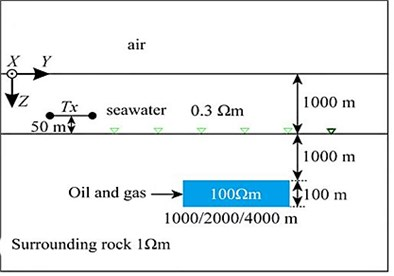a)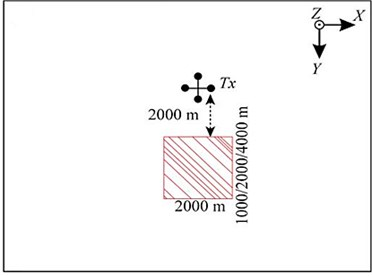b)

In the figure, the ${E}_{x}$, ${H}_{y}$, and ${H}_{z}$ curves are the results of the source arranged in the $X$ direction, and the ${E}_{Y}$, ${E}_{Z}$, ${H}_{X}$ curves are the source arranged in the $Y$ direction. Result. It can be seen from the figure that the amplitude curve and phase curve overlap very well. The entire simulation area is 100 km×100 km×100 km (taking a example of a 2 km×2 km oil and gas reservoir model.

The initial grid is shown in Fig. 9(a). Although the grid area is very large, the cells near the outer boundary are very large; the area of interest in the extracted grid is displayed separately as shown in Fig. 9(b) (0 km $ 1 km, -0.5 km $ 8.5 km, -0.5 km $ 2.5 km).

Fig. 8Comparison of adaptive finite element solution with finite volume method (3D oil & gas model)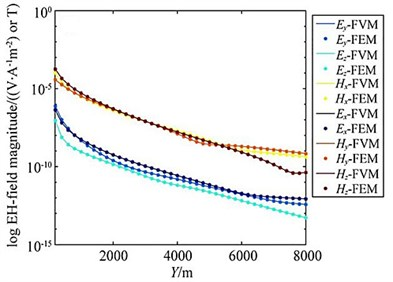a)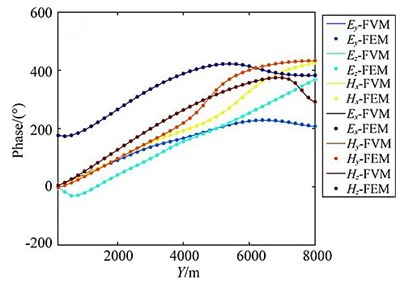b)

Fig. 9Grid schematic (3D oil and gas model)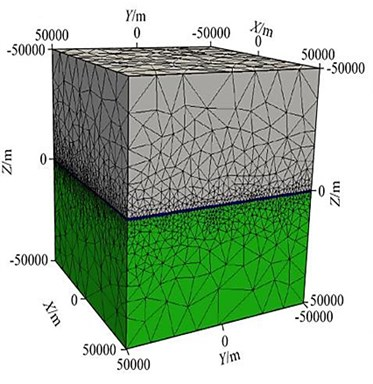a)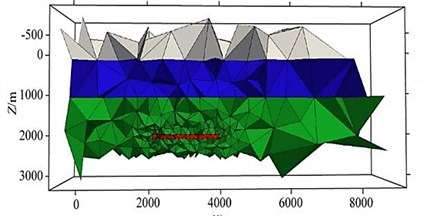b)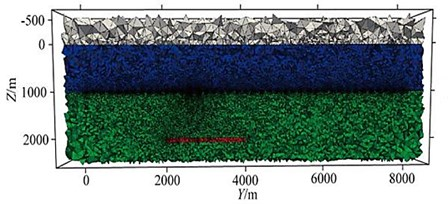c)

It can be seen that the initial grid is very rough, and there is no refinement of the grid of the emission source, the receiving point and the oil and gas reservoir area; after 15 grid refinements, it converges, and the grids in some areas the refinement is shown in Fig. 9(c), and there is good encryption near the receiving point and the oil and gas reservoir area, which ensures the accuracy of the numerical solution.

Analysis of the electric field curve of the horizontal thin plate-shaped high-resistance oil and gas reservoir model found that only when its horizontal size is at least 2 times the buried depth, can it be distinguished by CSEM. At present, the commonly used qualitative interpretation method of marine CSEM data it is to calculate the ratio of the electromagnetic vibration spectrum field amplitude of the reservoir containing oil and gas; to the electromagnetic vibration spectrum field amplitude of the background model using the reservoir without oil and gas, called the normalized field. Taking the interference factors such as observation error and noise into account, calculate the electromagnetic vibration spectrum field amplitude of the target model and the background model the ratio of the difference to the background noise is used to measure the size of the anomaly, which is called the effective anomaly. The calculation formula is:

21
$\mathrm{\Delta }=\frac{\left|\left|{E}_{M}\right|-\left|{E}_{O}\right|\right|}{{E}_{noise}},$
22
${E}_{noise}=\sqrt{{E}_{rel}^{2}+{E}_{rot}^{2}+{E}_{abs}^{2}},$

among them, $\left|{E}_{M}\right|$ is the electric field amplitude of the reservoir model containing oil and gas; $\left|{E}_{o}^{}\right|$ is the electric field amplitude of the background model without oil and gas reservoirs; $\left|{E}_{noise}\right|$ is electric field noise; ${E}_{rel}$ is the relative error of the amplitude, including the error generated by the instrument system and the observation error; ${E}_{abs}$ is the absolute error, is the noise floor of the transmitting-receiving system, usually taken ${E}_{abs}=1{0}^{-16}V\cdot {A}^{-1}{m}^{-2}$; ${E}_{rot}$ it is the error caused by the rotation angle of the receiving station, and the rotation angle error is taken here as 5°. Figs. 10(a) and 10(b) are the different lengths in the marine oil and gas reservoir model (Fig. 7) (the length in the $Y$ direction is 0 m respectively (that is, the oil and gas reservoirs are not included). (1 km, 2 km, 4 km) of the horizontal electric field EY amplitude curve and effective abnormal curve, Fig. 10(c) and 10(d) correspond to the amplitude curve and effective abnormal curve of the vertical electric field EZ respectively. It can be seen that if you only look at the amplitude curve, it is difficult to distinguish the anomaly of the horizontal electric field with a length of 1 km and 2 km in the oil and gas reservoir, but the anomaly in the effective anomaly curve (Fig. 10(b), 10(d)) is more obvious, especially the effective anomaly calculated by the vertical electric field, which is high at both ends of the oil and gas reservoir. The anomaly reflects, and the effective anomaly takes into account the influence of the data observation error and the transmitter attitude error on the electromagnetic vibration spectrum field response. The anomaly resolution is clearer and more effective. The longer the oil and gas reservoir length, the larger the effective anomaly .

Fig. 10a), c) Horizontal electric field amplitudes and b), d) effective anomalies of different oil and gas reservoirs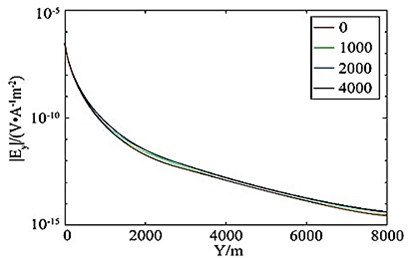a)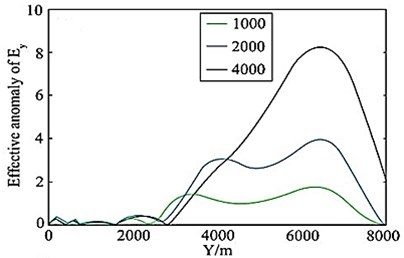b)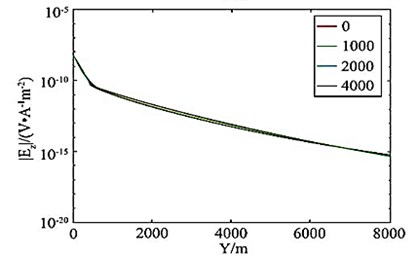c)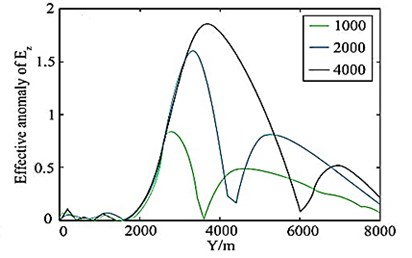d)

Table 1Mesh refinement parameters

 Refinement times Number of units Edge number CPU time Time to solve the equation/s Time spent per field/s Occupied memory/G Relative error RMS 1 93038 110704 85.93 10.02 66.22 1.371 2 146130 172236 147.83 27.60 104.83 2.776 33.3 3 214370 251286 222.47 54.80 145.41 4.681 20.8 4 308725 360558 347.73 119.43 196.05 8.025 14.4 5 455001 530080 557.36 226.58 282.89 12.858 5.5 6 633025 736331 888.07 460.58 360.14 20.589 3.5 7 864621 1004659 1339.38 765.34 480.98 30.426 2.4 8 1096976 1273416 1777.38 1167.96 491.31 41.595 1.5 9 1382474 1603859 2566.78 1824.59 591.07 57.133 1.2 10 1773893 2057286 4233.84 3085.89 846.37 81.037 0.9

### 4.3. Three-dimensional tilt anomaly model

Fault fracture zones, contact zones and metal veins can often be approximated by inclined plates. Establish a three-dimensional land model as shown in Fig. 11: the air resistivity is 108 Ωm, and there is an angle in the uniform half-space model with the resistivity of 100 Ωm.

A 45° inclined plate-shaped body, with the top exposed on the ground, with a resistivity of 30 Ωm. The three-dimensional scale of the plate-shaped body is 200 m×3000 m×1500 m, and the distribution range along the $X$ direction: the width is 200 m, the top is [750, 950] m, and the bottom it is [–950, –750] m; along the $Y$ direction, the distribution range is [5500, 8500] m, and the length is 3000 m. On the ground ($Z=$0 m), $Y=$5 km, 6 km, 7 km, 8 km, 9 km are arranged respectively. There are 205 measuring points in total, each measuring line is 2 km in length, containing 41 measuring points, and the interval is 50 m. The length of the grounding long wire source is 1km, and the center is placed at (0, 0, 0), along $X$ placed in the direction, the emission current is 1 A, and the emission frequency is sampled at logarithmic intervals between –1 and 1000 Hz, with a total of 11 sampling points [33, 34].

Fig. 11Land model of inclined plate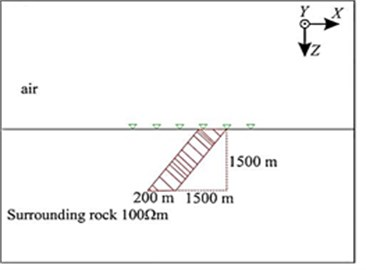a)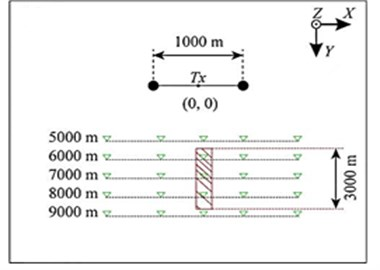b)

The calculation area of this example is 60 km×60 km×60 km, and the area of interest in the grid is extracted and displayed separately as shown in Fig. 12 (0 km $ 2 km, 4.5 km $ 9.5 km, 0 km $ 2 km). The mesh section is shown in Fig. 12(a). After 10 iterations, it converges (take 10 Hz as an example). At this time, the mesh has little effect on the change of the result. The final mesh section is shown in Fig. 12(b). There is good encryption everywhere.

Fig. 12Part grid diagram (land model)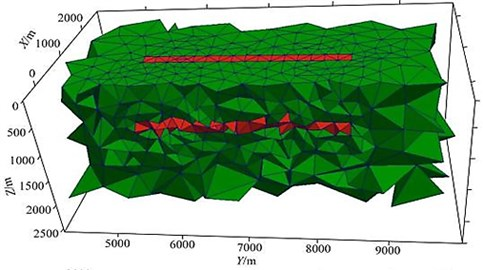a)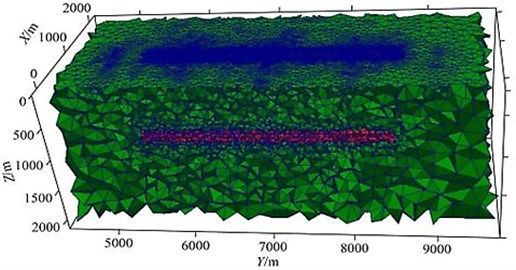b)

Fig. 13 shows the apparent resistivity slice of Kania.

Fig. 13Carnia apparent resistivity 3D slice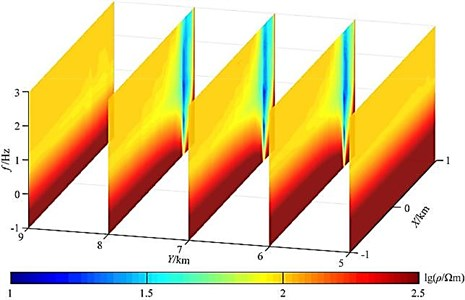No oblique plate anomaly can be seen on the 5 km and 9 km survey lines, and it can be clearly seen on the 6 km, 7 km and 8 km survey lines. Low resistance is abnormal. The apparent resistivity profiles of the three slices are basically the same. At 750-950 m of the exposed surface area, due to the influence of static effects, there is a phenomenon of noodles in the apparent resistivity, and further inversion is needed to better determine the tilt the inclination of the slab body, and a clearer resistivity interface can still be seen between -750 and 750 m. This part of the abnormality is the influence of the inclined slab body. In order to verify the correctness of the results, especially the high frequency in the case of the results, we compared the profile with the 2D adaptive finite element algorithm at the 7 km survey line, and the results are basically the same, as shown in Fig. 14.

Fig. 14Y= 7 km slice of apparent resistivity of the line of measurement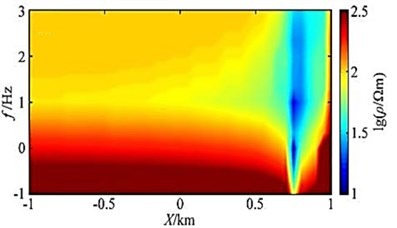a)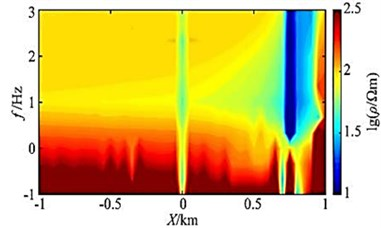b)

In the high frequency band (100-1000 Hz), within the range of [-1 km, 0] the apparent resistivity is all 100 Ωm, thus verifying the correctness of the high-frequency results. On the apparent resistivity slice, we can see a more obvious oblique plate-like body abnormality, and there is a phenomenon of noodles at 750-950 m. However, the 2D slice reflects the infinitely extending sloping plate-shaped body in the $Y$ direction, the apparent resistivity is low and the abnormal value is lower, and there is a large calculation error near the source due to the influence of the algorithm.

## 5. Conclusions

This article uses an adaptive vector finite element algorithm is used to realize the CSEM3D forward simulation and the primary/secondary field separation algorithms are utilized for the calculation of the electromagnetic vibration spectrum field response. The adaptive mesh refinement algorithm based on posterior error estimation refines the mesh, reduce the singularity of the source point, and improve the numerical accuracy of the electromagnetic vibration spectrum field near the field source and the receiving point. High-quality finite element solution is obtained using unstructured tetrahedral element grid method for discretization of geoelectric model, while posterior error estimation method is used to guide the grid encryption. The algorithms utilized can calculate the electromagnetic vibration spectrum field of a galvanic source with a finite length in any posture, and the source and receiving points can be placed in any position, which has good versatility.

This article establishes a layered oil and gas model and the analytical solutions are compared and the relative error of horizontal electromagnetic vibration spectrum field component amplitude is observed less than 1 % and the phase difference of 1° is observed. It is revealed from the numerical simulation and analysis that for the vertical electromagnetic vibration spectrum field component, when the transmission and reception distance is small (less than 5 km), the amplitude the relative error is less than 1 %, and the phase difference is within 1°. However, when the transmission distance is greater than 5 km, the relative amplitude error is larger, and the maximum phase difference of the ${E}_{Z}$ component is 1.4. this experimentation verifies the correctness and accuracy of the algorithm.

The correctness of the proposed algorithm is further verified by establishing a horizontal thin plate-shaped high-resistance oil and gas reservoir model. The three-dimensional forward modelling algorithm of ocean controllable source electromagnetic vibration spectrum based on staggered grid finite volume discretization are compared and it was analysed that the amplitude and phase coincide very well. It was found that the effective anomaly can better reflect the high-resistance oil and gas compared to the amplitude curve. For the established land model, the apparent resistivity of the 5 survey lines is calculated. It was revealed from the outcomes that there is an obvious static effect at the surface outcrop, and further inversion is needed to better determine the position and inclination angle of the inclined plate.

25 April 2021
Accepted
20 May 2021
Published
30 September 2021
Keywords
controllable source electromagnetic vibration spectrum
three-dimensional performance